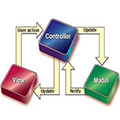# 【抽奖平台开发（4）】基于MVC模式实现数据后台管理操作的可视化（PHP+HTML+MySQL）★ 工程实战 同时被 3 个专栏收录27 篇文章 4 订阅#### 一、后台MVC开发模式的选择及开发思路MVC开发模式运用到抽奖平台后台开发上来，如上图所示。用户通过浏览器访问index.php进入Controller层，在Controller层的Controller.php文件中，定义一个基于用户不同请求（查看抽奖列表、中奖详情、删除数据）调用相对应模型后返回视图页面的控制器。此后则是进入Model层通过Model.php实现数据的操作。数据的操作除了命令语句的发出，还需要数据库的连接与响应，这一步骤就交给BaseModel.class.php（基础模型类）和MySQLDB.class.php（mysql数据库操作类）进行处理，此外，为保证系统中一个类只有一个实例，通过ModelFactory.class.php完成单例工厂的搭建以实现模型类的单例。最后Controller层的控制器调用与用户请求相对应的模型实现用户的操作请求后将通过View层返回视图页面，即View-index.html（“显示抽奖列表”、“删除抽奖信息”操作的视图返回页面）与View-GiftInfo.html（“查看抽奖结果详情”操作的视图返回页面）。

/www/wwwroot/***.com
└── 后台
├── index.php
├── Framework
│   ├── ModelFactory.class.php
│   ├── BaseModel.class.php
│   └── MySQLDB.class.php
└── Application
├── Controller
│   └── Controller.php
├── Model
│   └── Model.php
└── Views
├── View-index.html


#### 二、基于后台需求修复前台缺陷

（由于我在前台并未设置抽奖用户信息录入页面，因此不传输抽奖用户信息，其实现方法是设计一个抽奖用户信息录入页面，通过表单形式将录入信息提交至PHP即可，十分简单，故也不再多述了）

###### 1. 重新配置数据库结构###### 2. 在前台PHP中获取“中奖时间”和“中奖内容”
<?php
$tm = date('Y-m-d H:i:s',time());$results=$_GET['results']; echo "Time:" .$tm ."<br>";
echo "Gift lists:" . $results ."<br>"; ?>  ###### 3. 存储至数据库 <?php header("content-type:text/html;charset=utf-8"); @$db=mysqli_connect("localhost","用户名","密码","数据库名称");
if(mysqli_connect_errno()){
echo("Error:Couldnot connect the database");
exit;
}
/在获取“中奖时间”和“中奖内容”后：
$strsql = "insert into gift(times,gift) values('$tm',$results)";$result=mysqli_query($db,$strsql);
if(!$result){ echo("fail to insert data"); }else{ echo("sucess in insert data"); } @ mysqli_free_result($result);
mysqli_close($db); ?>  ###### 4. 测试#### 三、平台共用部分（Framework）开发 在明晰开发思路与文件结构后，我们正式开始后台的搭建工作。首先是整个平台公共部分的开发。根据开发思路，为保证系统中一个类只有一个实例，通过ModelFactory.class.php完成单例工厂的搭建以实现模型类的单例；此外由于用户的所有操作是基于数据库信息管理的操作，这一套步骤由BaseModel.class.php（基础模型类）和MySQLDB.class.php（mysql数据库操作类）完成。 ###### 1. ModelFactory.class.php 为防止重复实例化，减少消耗系统和内存的资源，有且仅有一个实例对象，我们常选择单例模式。所谓单例模式，是一种常用的软件设计模式，在它的核心结构中只包含一个被称为单例的特殊类。单例模式会阻止其他对象实例化其自己的单例对象的副本，从而确保所有对象都访问唯一实例。由于在Model层我们无法保证模型类本身是单例，因此有必要设计出一个“单例工厂类”，通过该单例工厂类，去“获取”模型类的类名，并返回给类的一个实例（对象），以确保单例。 <?php class ModelFactory{ Static$all_model = array();						//用于存储各个模型类的唯一实例（单例）
Static function M( $model_name ){ //$model_name是一个模型类的类名
if( !isset(static::$all_model[$model_name])							//如果不存在
||
!( static::$all_model[$model_name] instanceof  $model_name ) //或不是其实例 ) { static::$all_model[$model_name] = new$model_name();
}
return static::$all_model[$model_name];
}
}

###### 2. MySQLDB.class.php（mysql数据库操作类）

（1）执行任意的增删改语句
（2）执行返回一行/多行/一个数据（用于计数）的“查询语句”（分别返回“一维数组”、“二维数组”、“数据值”）
（3）执行任何sql语句，并进行错误处理，或返回执行结果

<?php
class MySQLDB{
private $link = null; //用于存储连接成功后的“资源” private$host;
private $port; private$user;
private $pass; private$charset;
private $dbname; private static$instance = null;
static  function GetInstance($config){ if( !(self::$instance instanceof self) ){
self::$instance = new self($config);
}
return self::$instance; } private function __clone(){} private function __construct($config){
$this->host =$config['host'];
$this->port =$config['port'];
$this->user =$config['user'];
$this->pass =$config['pass'];
$this->dbname =$config['dbname'];
$this->charset = !empty($config['charset']) ? $config['charset'] : "utf8" ;$this->link  =  mysqli_connect("{$this->host}:{$this->port}", "{$this->user}", "{$this->pass}","{$this->dbname}") or die("连接失败"); } //这个方法实现连接关闭 function closeDB(){ mysqli_close($this->link);
}
//这个方法为了执行一条增删改语句，它可以返回真假结果。
function exec($sql){$result = $this->query($sql);
return true;
}
//这个方法是返回一行数据的“查询语句”，它可以返回一维数组
function GetOneRow($sql){$result = $this->query($sql);
$rec = mysqli_fetch_assoc($result );//取出第一行数据（其实应该只有这一行）
mysqli_free_result( $result ); //提前释放资源（销毁结果集），否则需要等到页面结束才自动销毁 return$rec;
}
//这个方法是返回多行数据的“查询语句”，它可以返回二维数组
function GetRows($sql){$result = $this->query($sql);
$arr = array(); //空数组，用于存放要返回的结果数组（二维） while ($rec = mysqli_fetch_assoc( $result ) ){$arr[] = $rec; //此时，$arr就是二维数组了！
}
mysqli_free_result( $result ); return$arr;
}
//这个方法是返回一个数据的“查询语句”，它可以返回一个直接值
function GetOneData($sql){$result = $this->query($sql);
$rec = mysqli_fetch_row($result );
$data =$rec;
mysqli_free_result( $result ); return$data;
}
//统筹上面的所有方法，用于执行任何sql语句，并进行错误处理，或返回执行结果；
private function query( $sql ){$result = mysqli_query($this->link,$sql);
if( $result === false){ echo "<p>sql语句执行失败，请参考如下信息："; echo "<br />错误代号：" . mysql_errno(); echo "<br />错误信息：" . mysql_error(); echo "<br />错误语句：" .$sql;
die();
}
return $result; //返回的是“执行结果” } } ?>  ###### 3. BaseModel.class.php（基础模型类，现阶段仅需用于存储数据库账号密码） <?php class BaseModel{ //用于存储数据库工具类的实例（对象） protected _dao = null; function __construct(){$config = array(
'host' => "localhost",
'port' => 3306,
'user' => "用户名",
'pass' => "密码",
'charset' => "utf8",
'dbname' => "数据库名称"
);
$this->_dao = MySQLDB::GetInstance($config);
}
}


#### 四、Controller层开发（PHP）

/www/wwwroot/***.com
└── 后台
├── index.php
└── Application
└── Controller
└── Controller.php

###### 1. index.php：

<?php
require './Framework/MySQLDB.class.php';
require './Framework/ModelFactory.class.php';
require './Framework/BaseModel.class.php';
require './Application/Modelss/Model.php';
require './Application/Controllers/Controller.php';

$ctrl = new Controller();$act = !empty($_GET['act']) ?$_GET['act'] : "Index";
$action =$act . "Action";
$ctrl->$action();	//可变函数————>>可变方法
?>

###### 2. Controller.php：

<?php
class Controller{
//显示抽奖列表
function IndexAction(){
$obj_List = ModelFactory::M('ListModel');$data1 = $obj_List->GetAllList(); //是一个二维数组$data2 = $obj_List->GetListCount(); //是一个数字 include './Application/Views/View-index.html'; } //删除指定抽奖信息 function DelAction(){$id = $_GET['id'];$obj = ModelFactory::M('ListModel');
$result =$obj->delListById($id); echo "<script>alert('删除成功！')</script>"; echo "<meta http-equiv='Refresh' content='0;URL=index.php'>"; } //查看指定抽奖结果详情 function DetailAction(){$id = $_GET['id'];$obj = ModelFactory::M('ListModel');
$data =$obj->GetGiftInfoById($id); include './Application/Views/View-GiftInfo.html'; } }  在这里需要额外补充强调几点： 1. index.php中的语句含义：代替了Controller.php内所有if判断逻辑，即： if(!empty($_GET['act']) && $_GET['act'] == 'detail'){ DetailAction(); } else if(!empty($_GET['act']) && $_GET['act'] == 'del'){ DelAction(); } else{ IndexAction(); }  1. 载入视图文件的逻辑起点根据文件结构，Controller.php需要回退至Application文件夹后再进入Views文件夹最后才能载入视图文件，但是需要注意的是，所有逻辑起点都应当从index.php开始，所以不需要考虑要回退多少个目录，加多少个"."。 1. Controller.php中删除操作后的跳转问题 echo "<script>alert('删除成功！')</script>"; echo "<meta http-equiv='Refresh' content='0;URL=index.php'>";  在删除数据成功后，页面自动跳转回入口页面，目的一方面在于保障数据结构安全，另一方面在于回归逻辑层面的初始状态，保障操作逻辑的完整与统一。 1. $_GET安全性问题

$data =$this->_dao->GetRows($sql); return$data;
}
function GetListCount(){
$sql = "select count(*) as c from gift;";$data = $this->_dao->GetOneData($sql);
return $data; } function delListById($id){
$sql = "delete from gift where id =$id;";
$data =$this->_dao->exec($sql); return$data;
}
function GetGiftInfoById($id){$sql = "select * from gift where id = $id;";$data = $this->_dao->GetOneRow($sql);
return $data; } }  其中GetRows($sql)GetOneData($sql)GetOneData($sql)exec($sql)是MySQLDB工具类的方法，_dao则是从父类BaseModel$_dao属性继承而来的用于存储的对象，是MySQLDB工具类的实例。#### 六、Views层开发（HTML）

###### 1. View-index.html
<!DOCTYPE html PUBLIC "-//W3C//DTD XHTML 1.0 Transitional//EN" "http://www.w3.org/TR/xhtml1/DTD/xhtml1-transitional.dtd">
<html xmlns="http://www.w3.org/1999/xhtml" xml:lang="zh-cn">
<meta http-equiv="Content-Type" content="text/html;charset=UTF-8" />
<title>抽奖结果查询后台</title>
<meta name="keywords" content="抽奖结果查询后台" />
<meta name="description" content="抽奖结果查询后台" />
<meta name="viewport" content="width=device-width, initial-scale=1.0, maximum-scale=1.0, user-scalable=0">
<style type="text/css">
.t1{color:red;}
.t2{background:#cccccc}
</style>
<script type="text/javascript"></script>
<body>
<div style="position: absolute;left: 50%;transform:translateX(-50%);white-space:nowrap" >
<div style="font-size: 23px;font-weight: bold;text-align: center">抽奖结果</div>
<br />
<div  class="fr">
<table border='1'>
<tr>
<th >编号</th>
<th >中奖时间</th>
<th >奖品内容</th>
<th >数据管理</th>
</tr>
<?php
foreach( $data1 as$key => $rec ) { ?> <tr> <td ><?php echo$rec['id']; ?></td>
<td class='t1'><?php echo $rec['times']; ?></td> <td class='t2'><?php echo$rec['gift']; ?></td>
<td>
<a href='?act=del&id=<?php echo $rec['id']; ?>' οnclick='return queren()'>删除</a> <a href='?act=detail&id=<?php echo$rec['id']; ?>' >详细</a>
</td>
</tr>
<?php
}
?>
</table>
<br />
当前抽奖记录总数：<?php echo $data2;?> </div> </div> </body> </html> <script> function queren(){ return window.confirm("是否确认删除？"); } </script> <script src="https://code.jquery.com/jquery-1.9.1.min.js"></script> <script>$(function(){
setInterval(aa,1000);
function aa(){
$(".fr").load(location.href+" .fr"); } }) </script>  对于用户的抽奖结果，后台应当能够实时检测，手动刷新以同步数据库信息的方式显然不符合管理需求，对此实现方式大致有二，其一，设置定时器自动刷新页面以同步数据（实现方式见下方代码块）；其二，不刷新页面更新数据，对于用户量小的平台可以基于Ajax实现轮询，用户量大可选择长轮询或workman Socket服务器框架。 <script> function fresh_page(){ window.location.reload(); }; setTimeout('fresh_page()',10000); </script>  具体应用到本后台的搭建中，由于在Views层是通过<?php echo$rec['**']; ?>的形式更新数据，因此并不一定需要使用Ajax轮询，综合考虑页面整体刷新的局限性，在这里我选用了jQUery的局部刷新方法，将需要同步的内容包装在class="fr"div标签中，通过div的局部刷新以实时同步数据库信息至后台：

<div  class="fr">
//需要同步的内容
</div>

<script src="https://code.jquery.com/jquery-1.9.1.min.js"></script>
<script>
$(function(){ setInterval(aa,1000); function aa(){$(".fr").load(location.href+" .fr");
}
})
</script>###### 2. View-GiftInfo.html
<!DOCTYPE html PUBLIC "-//W3C//DTD XHTML 1.0 Transitional//EN" "http://www.w3.org/TR/xhtml1/DTD/xhtml1-transitional.dtd">
<html xmlns="http://www.w3.org/1999/xhtml" xml:lang="zh-cn">
<meta http-equiv="Content-Type" content="text/html;charset=UTF-8" />
<title>详细信息</title>
<meta name="keywords" content="详细信息" />
<meta name="description" content="详细信息" />
<meta name="viewport" content="width=device-width, initial-scale=1.0, maximum-scale=1.0, user-scalable=0">
<style type="text/css">
</style>
<script type="text/javascript"></script>
<body>
<div style="position: absolute;left: 50%;transform:translateX(-50%)" >
<div style="font-size:23px;font-weight:bold;white-space:nowrap">关于编号<font color=red><?php echo $data['id'] ?></font>的详细信息如下：</div> <hr /> <nobr />中奖时间：<?php echo$data['times'] ?>
<br/>
<br/>
<br/>
<br/>
<nobr />奖品价格：<?php echo '信息暂未录入' ?>
<br/>
<br/>
<nobr />中奖者姓名：<?php echo '信息暂未录入' ?>
<br/>
<br/>
<nobr />中奖者联系方式：<?php echo '信息暂未录入' ?>
<hr />
<a href='?'>返回</a>
</div>
</body>
</html>#### 七、测试：1. 数据操作之“删除数据”2. 查询“奖品详细信息”10-20
01-21162
01-28876
03-03
08-181万+
04-201640
01-181万+
01-231万+
05-3198
03-152万+
03-151万+
03-152万+
04-283万+点击重新获取扫码支付余额充值